## SOCNET@LISTS.UFL.EDU

#### View:

 Message:[First|Previous|Next|Last] By Topic:[First|Previous|Next|Last] By Author:[First|Previous|Next|Last] Font: Proportional Font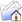LISTSERV Archives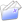SOCNET HomeSOCNET February 2011

Subject:search for complete invariants for digraphs

From:Date:Wed, 23 Feb 2011 22:54:25 +0100

Content-Type:text/plain

Parts/Attachments: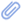text/plain (67 lines)
 ```***** To join INSNA, visit http://www.insna.org ***** > Tad, do you imply that a solution is known for k = 1? Isn't this NP? > --Moses Moses, what is NP? As regards k, do you mean the number of parameters in a complete collection of invariants? Basically, k is unimportant, but the smaller, the better. It would be nice to have for digraphs something similar to 'dimension' for 'finite-dimensional real vector spaces', where dimension is a complete invariant, that is, any two vector spaces having the same dimension are isomorphic, actually, all n-dimensional real vector spaces are isomorphic with R^n. For digraphs, the simplest structural parameters are the number p of points and the number q of arcs. Of course, these two do not suffice to determine a digraph 'uniquely up to isomorphism'. What other parameters should be added? That is the problem, to my (limited) knowledge, still unsolved. Tad >> >> Dear Socnet Members >> >> Let me address a  question to those of you who have been watching >> developments  in GRAPH THEORY. >> >> Is the following statement true? >> >> 'No nontrivial complete set collection of invariants has yet been found >> for directed graphs >> >> Definitions >> >> A (finite) DIRECTED GRAPH is a mathematical object of the form G=(X,R), >> where X is a finite set (of points) and R is  a binary relation in X. >> >> Two digraphs G=(X,R) and G =(X ,R ) are ISOMORPHIC if there exists a 1-1 >> mapping F of X onto X  such that xRy if and only if  F(x)R F(y), for any >> x,y in X. >> >> A STRUCTURAL PARAMETER of a digraph is any mapping P which assigns a >> numerical value to any digraph in such a way that  P(G)=P(G ) if G and G >> are isomorphic. >> >> A COMPLETE SET OF INVARIANTS is any finite collection P1, ,Pk of >> structural parameters such that, for any two digraphs G and G , the >> equations  P1(G)=P1(G ), ,Pk(G)=Pk(G ) imply that G and G  are >> isomorphic. >> >> For any n, let  gn denote the number of equivalence classes of  the >> isomorphism relation in  the set of digraphs with X={1, ,n}. The numbers >> 1, ,gn can be used as  code symbols of equivalence classes. A 'trivial' >> set of invariants is obtained by assigning to any G=(X,R) the number n >> of >> points (n=|X| ) and an appropriate symbol from the range from 1 to gn. >> Hence, by saying that a set of invariants is  NONTRIVIAL I mean that >>  the >> values of P1, ,Pk for two n-point digraphs G and G   can be computed >> without prior knowledge of the list of isomorphism classes. >> >> Tad Sozanski >> www.cyf-kr.edu.pl/~ussozans/ >> _____________________________________________________________________ SOCNET is a service of INSNA, the professional association for social network researchers (http://www.insna.org). To unsubscribe, send an email message to [log in to unmask] containing the line UNSUBSCRIBE SOCNET in the body of the message.```

#### Search Archives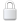Log In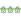Get Password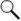Search Archives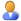Subscribe or Unsubscribe